# Plotting Tracers on Horizontal Planes (Depth Slices)¶

This notebook contains discussion, examples, and best practices for plotting tracer variable (e.g. temperature, salinity, sea surface height) results from NEMO as colour meshes. It extends the discussion of horizontal slice visualizations in the Plotting Bathymetry Colour Meshes.ipynb notebook with plotting of slices at selected depths and time steps of variables like temperature and salinity that are calculated on the 3D grid. Topics include:

• Reading tracer variable values from NEMO *grid_T.nc results files
• Plotting sea surface height fields at selected times
• Approximate land areas masks for tracer field plots
• Using slices to zoom in on domain regions
• Plotting contour bands and lines
• Plotting temperature fields at selected depths
• Adding contour lines to colour mesh plots
• Anomaly plots
• Plotting salinity fields with various colour scales

As always, we'll start with our imports, and activation of the Matplotlib inline backend so that our plots will appear below the cells that create them.

In :
import matplotlib.pyplot as plt
import netCDF4 as nc
import numpy as np

from salishsea_tools import (
nc_tools,
viz_tools,
)

In :
%matplotlib inline


## NEMO grid_T Results Files¶

In NEMO, scalar quantities like temperature, salinity, are referred to as tracers. They and sea surface height (pressure) are stored at the tracer grid point locations of an Akawara C grid which NEMO calls the T-grid.

The results files that contain the scalar values for a run have names like:

SalishSea_1h_20021026_20021026_grid_T.nc


where:

• The SalishSea part comes from the "experience name" variable cn_exp in the &namrun namelist
• The 1h indicates both the time interval of the results in the file and the time period over which the values are averaged. In the example above the values are hourly averages that are reported hourly. The time interval for a given run is specified in the iodef.xml file that is used for the run.
• The date parts, 20021026 twice in the example above, are the start and end dates of the run.
• The grid_T part indicates that the file contains T-grid variable results. The particular variables stored for a given run are specified in the iodef.xml file that is used for the run.

So, the results file above contains hourly averaged values of T-grid variables for the single day run for 2002-10-26.

We'll use a results file from one of the spin-up runs that are stored in the /results/SalishSea/spin-up/2002/ directory. Let's load the file into a netCDF dataset object and look at its dimensions:

In :
tracers = nc.Dataset('/results/SalishSea/spin-up/2002/26oct/SalishSea_1h_20021026_20021026_grid_T.nc')

In :
nc_tools.show_dimensions(tracers)

<class 'netCDF4._netCDF4.Dimension'>: name = 'x', size = 398

<class 'netCDF4._netCDF4.Dimension'>: name = 'y', size = 898

<class 'netCDF4._netCDF4.Dimension'>: name = 'deptht', size = 40

<class 'netCDF4._netCDF4.Dimension'> (unlimited): name = 'time_counter', size = 24

<class 'netCDF4._netCDF4.Dimension'>: name = 'tbnds', size = 2



As is the case for most NEMO results files, the dataset has:

• The 2 lateral dimensions that are the same as those of the bathymetry file (see the Exploring netCDF Files.ipynb notebook); x and y with sizes 398 and 898, respectively.
• A depth levels dimension that NEMO calls deptht with a size of 40.
• A time dimension that NEMO calls time_counter. It is marked as unlimited meaning that it is the dimension along which the file grows as NEMO calculates results. Its size in this dataset is 24 because the file contains 1 day's hourly results.
• A time counter bounds dimension called tbnds with a size of 2 that provides information about the time intervals in the time_counter dimension.

Now let's look at the variables in the dataset:

In :
nc_tools.show_variables(tracers)

odict_keys(['nav_lon', 'nav_lat', 'deptht', 'time_counter', 'time_counter_bnds', 'sossheig', 'votemper', 'vosaline', 'rain_rate', 'snow_rate'])


The first 5 variables correspond to the dataset's dimensions:

• nav_lon and nav_lat contain the longitudes and latitudes of lateral grid points (as we saw in the Exploring netCDF Files.ipynb notebook)
• deptht contains the depths of the vertical grid layers
• time_counter contains the model time values at the centre of each of the output intervals
• time_counter_bnds contains the start and end times of each of the intervals in time_counter

The remaining variables are calculated values from NEMO. Their names come from NEMO's (sometimes cryptic) "doctor" naming scheme:

• sossheig is the sea surface height field which has 2 spatial dimensions (y and x)
• votemper is the temperature field which has 3 spatial dimensions (y, x, and deptht)
• vosaline is the salinity field with the same 3 spatial dimensions
• rain_rate is the liquid precipitation rate field (2 spatial dimensions)
• snow_rate is the solid precipitation rate field (also 2 spatial dimensions). In the Salish Sea NEMO model all precipitation is constrained to be liquid, so the snow_rate values are always zero.

Taking a deeper look at the attributes and values of the time_counter and time_counter_bnds variables:

In :
nc_tools.show_variable_attrs(tracers, 'time_counter')
tracers.variables['time_counter'][:]

<class 'netCDF4._netCDF4.Variable'>
float64 time_counter(time_counter)
axis: T
standard_name: time
units: seconds since 2002-10-26 00:00:00
calendar: gregorian
title: Time
long_name: Time axis
time_origin:  2002-OCT-26 00:00:00
bounds: time_counter_bnds
unlimited dimensions: time_counter
current shape = (24,)
filling off


Out:
array([  1800.,   5400.,   9000.,  12600.,  16200.,  19800.,  23400.,
27000.,  30600.,  34200.,  37800.,  41400.,  45000.,  48600.,
52200.,  55800.,  59400.,  63000.,  66600.,  70200.,  73800.,
77400.,  81000.,  84600.])

The time values are measured in seconds since the date/time at which the run started, in this case midnight UTC on 2002-10-26. The values are the midpoints of the output intervals (1 hour in this case) because the calculated variable values in the output are averaged over the output interval.

Note that NEMO runs always start at midnight UTC on the date given in the &namrun namelist. It is coincidental that we are looking at a 2002-10-26 results file from the first day of a series of spin-up runs; spin-up runs for later dates also count time from midnight UTC on 2002-10-26, not from the beginning of the run period.

In :
nc_tools.show_variable_attrs(tracers, 'time_counter_bnds')
tracers.variables['time_counter_bnds'][:]

<class 'netCDF4._netCDF4.Variable'>
float64 time_counter_bnds(time_counter, tbnds)
unlimited dimensions: time_counter
current shape = (24, 2)
filling off


Out:
array([[     0.,   3600.],
[  3600.,   7200.],
[  7200.,  10800.],
[ 10800.,  14400.],
[ 14400.,  18000.],
[ 18000.,  21600.],
[ 21600.,  25200.],
[ 25200.,  28800.],
[ 28800.,  32400.],
[ 32400.,  36000.],
[ 36000.,  39600.],
[ 39600.,  43200.],
[ 43200.,  46800.],
[ 46800.,  50400.],
[ 50400.,  54000.],
[ 54000.,  57600.],
[ 57600.,  61200.],
[ 61200.,  64800.],
[ 64800.,  68400.],
[ 68400.,  72000.],
[ 72000.,  75600.],
[ 75600.,  79200.],
[ 79200.,  82800.],
[ 82800.,  86400.]])

The time_counter_bnds values are the start and end times of the output intervals. Although the variable attributes don't say so, it is evident that the values have the same units as the time values: seconds since midnight UTC on 2002-10-26.

We're going to use the time_counter variable values below to label plots, so let's alias it as timesteps:

In :
timesteps = tracers.variables['time_counter']


## Plotting Sea Surface Height Fields and Time Slicing¶

Looking at the attributes of the sossheig variable:

In :
nc_tools.show_variable_attrs(tracers, 'sossheig')

<class 'netCDF4._netCDF4.Variable'>
float32 sossheig(time_counter, y, x)
units: m
standard_name: sea surface height
_FillValue: 9.96921e+36
long_name: sea surface height
online_operation: ave(X)
interval_operation: 10.0
interval_write: 3600.0
coordinates: time_counter nav_lat nav_lon
unlimited dimensions: time_counter
current shape = (24, 898, 398)
filling off



sossheig is a 3-dimensional array. Its axes, (time_counter, y, x), reflect the netCDF CF1.6 conventional ordering, (t, z, y, x), with z excluded because there are not depth values. In particular, netCDF requires that the unlimited dimension be the leftmost one.

The shape is (24, 898, 398); i.e. 24 hours, 898 y-direction values, and 398 x-direction values.

The units, standard_name, and long_name attributes are self-explatory.

online_operation: ave(X) tells us that the values are averaged over the output interval. interval_write: 3600.0 tells us that the output interval is 3600 s = 1 hr. interval_operation: 10.0 tells us that the time step for the calculation of the values that are averaged is 10 s.

The _FillValue: 9.96921e+36 and filling off attribute values are clues that the sea surface height values are not masked (as we will see in a moment in a plot). In constrast to the bathymetry data file which contains metadata to automatically mask out land areas, we have to explicitly create land masks for NEMO output variables.

Let's alias that variable as ssh to save on keystrokes:

In :
ssh = tracers.variables['sossheig']


We can obtain the sea surface height field over the entire domain at a given time step by slicing on the first axis (remember that Python uses zero-based indexing):

In :
print(ssh.shape)
ssh

(898, 398)

Out:
array([[ 0.,  0.,  0., ...,  0.,  0.,  0.],
[ 0.,  0.,  0., ...,  0.,  0.,  0.],
[ 0.,  0.,  0., ...,  0.,  0.,  0.],
...,
[ 0.,  0.,  0., ...,  0.,  0.,  0.],
[ 0.,  0.,  0., ...,  0.,  0.,  0.],
[ 0.,  0.,  0., ...,  0.,  0.,  0.]], dtype=float32)

We can also slice on the 2nd and 3rd axes to obtain the field values over part of the domain:

In :
ssh_part = ssh[5, 250:325, 100:150]
print(ssh_part.shape)
ssh_part

(75, 50)

Out:
array([[ 0.        ,  0.        ,  0.        , ...,  0.        ,
0.        ,  0.        ],
[ 0.        ,  0.        ,  0.        , ...,  0.        ,
0.        ,  0.        ],
[ 0.        ,  0.        ,  0.        , ...,  0.        ,
0.        ,  0.        ],
...,
[-1.32869375, -1.324965  , -1.32320511, ...,  0.        ,
0.        ,  0.        ],
[-1.32933927, -1.32603157, -1.32355893, ...,  0.        ,
0.        ,  0.        ],
[-1.32961977, -1.32633412, -1.32388937, ...,  0.        ,
0.        ,  0.        ]], dtype=float32)

Plot a colour mesh of ssh at the first time step:

In :
fig, ax = plt.subplots(1, 1, figsize=(10, 8))
viz_tools.set_aspect(ax)
mesh = ax.pcolormesh(ssh)
fig.colorbar(mesh)

Out:
<matplotlib.colorbar.Colorbar at 0x7f9079ad8780>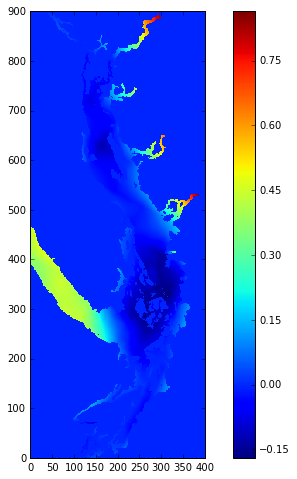As noted above, the values in NEMO results files are generally not masked like our bathymetry data. The land areas have zero as their value.

We can quickly create an approximate land mask for tracer variables by creating a NumPy Masked Array object with the zero values masked:

In :
ssh0 = np.ma.masked_values(ssh, 0)


Note, however, that creating the land mask that way will create land-like artifacts at grid points where the sea surface height happens to be within np.ma.masked_values()'s default absolute tolerance ($10^{-8}$) of zero. To be rigourously accurate for things like publication plots the land mask from the bathymetry data should be used.

That said, the quick land mask is adequate for most purposes and certainly improves the appearance of our plot:

In :
fig, ax = plt.subplots(1, 1, figsize=(10, 8))
viz_tools.set_aspect(ax)
mesh = ax.pcolormesh(ssh0)
fig.colorbar(mesh)

Out:
<matplotlib.colorbar.Colorbar at 0x7f90786fa940>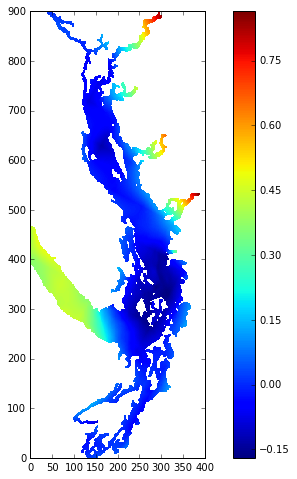We can use the same plotting techniques that we used for bathymetry in the Plotting Bathymetry Colour Meshes.ipynb notebook to set a colour for the land mask, tune the axes limits to remove white bands at the left and top edges, and add titles to the axes:

In :
fig, ax = plt.subplots(1, 1, figsize=(10, 8))
viz_tools.set_aspect(ax)
cmap = plt.get_cmap('jet')
mesh = ax.pcolormesh(ssh0, cmap=cmap)
cbar = fig.colorbar(mesh)
plt.axis((0, ssh0.shape, 0, ssh0.shape))

ax.set_xlabel('x Index')
ax.set_ylabel('y Index')
cbar.set_label('{label} [{units}]'.format(label=ssh.long_name.title(), units=ssh.units))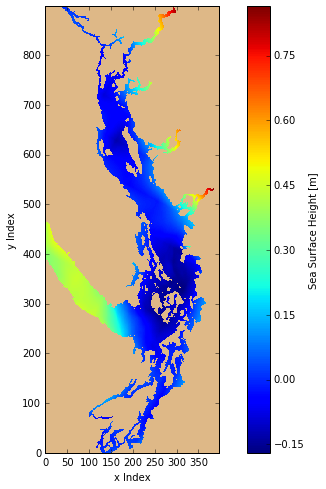Plotting the results from several time steps side by side takes just a little bit more work with the help of a couple of argument changes in plt.subplots(), a loop, and the Python built-in function zip():

In :
fig, axs = plt.subplots(1, 3, figsize=(16, 8), sharey=True)
cmap = plt.get_cmap('jet')

time_steps = (0, 3, 6)
for ax, t in zip(axs, time_steps):
viz_tools.set_aspect(ax)
mesh = ax.pcolormesh(ssh_t, cmap=cmap)
cbar = fig.colorbar(mesh, ax=ax)
ax.set_title('t = {:.1f}h'.format(timesteps[t] / 3600))
ax.set_xlabel('x Index')

axs.set_ylabel('y Index')
cbar.set_label('{label} [{units}]'.format(label=ssh.long_name.title(), units=ssh.units))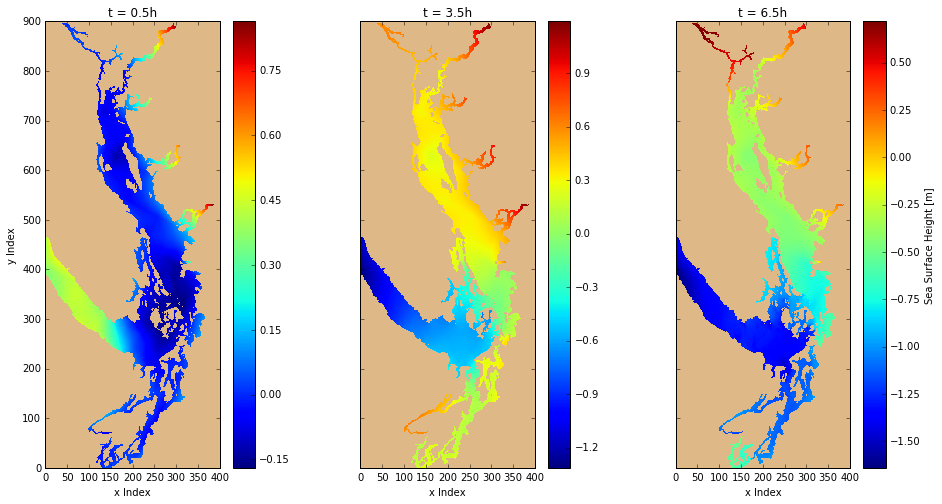Things to note in the above code cell:

• We're using the time_counter variable from the tracers dataset calculate the time in hours for the plot axes titles
• The first 2 arguments to plt.subplots() specify the number of rows and columns of axes objects to create within the figure object. The axes objects are returned as a tuple, axs in the code above.
• We increased the figure size to make room for the 3 axes and to give them enough space between to look good.
• The sharey=True argument to plt.subplots() causes all of the axes to have the same y-axis scale and suppresses the y-axis labels on all but the leftmost axes object.
• zip() returns, one at a time, tuples composed of the elements of the iterables given to it as arguments, in this case, the tuple of axes objects, axs, and the tuple of time step indices, time_steps. That allows us to associate each time step index with axes object in sequence, left to right.
• The ax=ax argument to fig.colorbar() is used to indicate with axes object in the figure the colour bar should be created for.
• The y-axis label is set on the leftmost axes object, axs.
• We take advantage of the fact that the value of cbar at the end of the loop is the rightmost colour bar and set its title after the loop has finished.

Once we've looked at the resulting set of plots we can use the vmin and vmax argument to ax.colormesh() to set the colour bar limits so that they are the same on all 3 plots and so that they encompass the sea surface height range of all 3:

In :
fig, axs = plt.subplots(1, 3, figsize=(16, 8), sharey=True)
cmap = plt.get_cmap('jet')

time_steps = (0, 3, 6)
for ax, t in zip(axs, time_steps):
viz_tools.set_aspect(ax)
mesh = ax.pcolormesh(ssh_t, cmap=cmap, vmin=-1.5, vmax=1)
cbar = fig.colorbar(mesh, ax=ax)
ax.set_title('t = {:.1f}h'.format(timesteps[t] / 3600))
ax.set_xlabel('x Index')

axs.set_ylabel('y Index')
cbar.set_label('{label} [{units}]'.format(label=ssh.long_name.title(), units=ssh.units))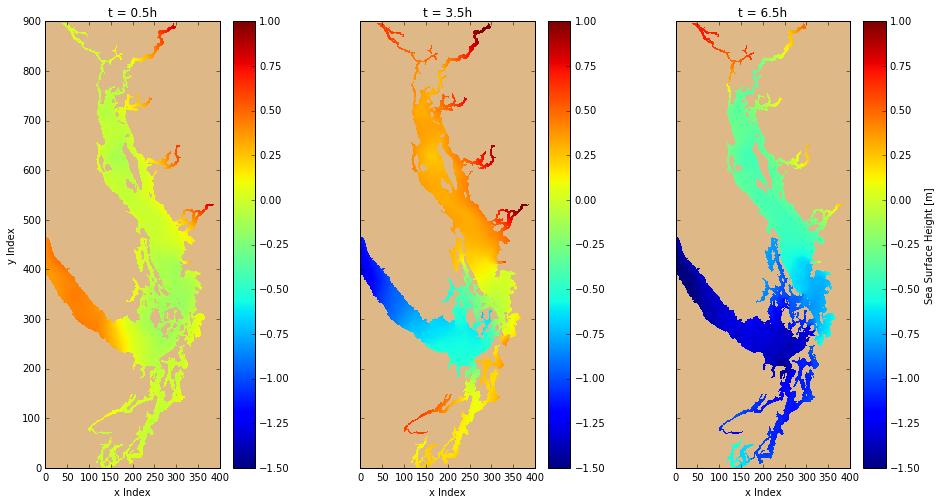## Zooming by Slicing¶

In the Plotting Bathymetry Colour Meshes.ipynb notebook we used the plt.axis() method to zoom in on a region of the domain. An alternative that is faster is to use slices on the y and x dimensions to plot the region of interest. That's faster because the plt.axis() technique comes only after a colour mesh has been calculated and then applies a display window to it. Using slices means that the colour mesh only has to be calculated for the region of interest. We could still use plt.axis() to tune the axes limits, or they can be set via a method call on the axes object, as can grid visibility.

In :
t = 3
y_slice = np.arange(250, 401)
x_slice = np.arange(200, 397)
ssh3_region = np.ma.masked_values(ssh[t, y_slice, x_slice], 0)

fig, ax = plt.subplots(1, 1, figsize=(10, 8))
viz_tools.set_aspect(ax)
cmap = plt.get_cmap('jet')
mesh = ax.pcolormesh(x_slice, y_slice, ssh3_region, cmap=cmap)
cbar = fig.colorbar(mesh, shrink=0.85)

ax.set_xlim(x_slice, x_slice[-1])
ax.set_ylim(y_slice, y_slice[-1])
ax.grid()

ax.set_xlabel('x Index')
ax.set_ylabel('y Index')
cbar.set_label('{label} [{units}]'.format(label=ssh.long_name.title(), units=ssh.units))
ax.set_title('t = {:.1f}h'.format(timesteps[t] / 3600))

Out:
<matplotlib.text.Text at 0x7f9076f5d978>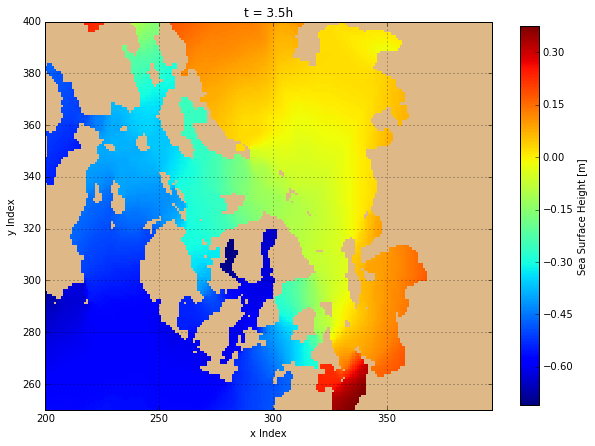## Plotting Contour Bands and Lines¶

The colour mesh field plots that we have created so far provide a view of the values plotted in which the colours vary continuously from one grid cell to the next. Sometimes it is preferrable to plot the values in distinct colour bands, especially if you are making quantitative assessments or comparisons. To achieve that we switch from plotting pseudocolour meshes to plotting contours, both as filled polygons, and as lines.

The axes.contourf() method creates filled polygons that we use to produce our colour bar in the same way as we did with the mesh collection returned by axes.pcolormesh(). The axes.contour() method adds contour lines at the poylgon edges and we add the collection of lines it returns to the colour bar with the cbar.add_lines() method.

Finally, we need to plot a contour line along the coastline to close the contour polygons. For that we need to use bathymetry data, and the viz_tools.plot_coastline() function allows us to draw the coastline on either grid or map coordinates.

In :
help(viz_tools.plot_coastline)

Help on function plot_coastline in module salishsea_tools.viz_tools:

plot_coastline(axes, bathymetry, coords='grid', isobath=0, xslice=None, yslice=None, color='black')
Plot the coastline contour line from bathymetry on the axes.

The bathymetry data may be specified either as a file path/name,
or as a :py:class:netCDF4.Dataset instance.
If a file path/name is given it is opened and read into a
:py:class:netCDF4.Dataset so,
if this function is being called in a loop,
it is best to provide it with a bathymetry dataset to avoid

:arg axes: Axes instance to plot the coastline contour line on.
:type axes: :py:class:matplotlib.axes.Axes

:arg bathymetry: File path/name of a netCDF bathymetry data file
or a dataset object containing the bathymetry data.
:type bathymetry: str or :py:class:netCDF4.Dataset

:arg coords: Type of plot coordinates to set the aspect ratio for;
either :kbd:grid (the default) or :kbd:map.
:type coords: str

:arg isobath: Depth to plot the contour at; defaults to 0.
:type isobath: float

:arg xslice: X dimension slice to defined the region for which the
contour is to be calculated;
defaults to :kbd:None which means the whole domain.
If an xslice is given,
a yslice value is also required.
:type xslice: :py:class:numpy.ndarray

:arg yslice: Y dimension slice to defined the region for which the
contour is to be calculated;
defaults to :kbd:None which means the whole domain.
If a yslice is given,
an xslice value is also required.
:type yslice: :py:class:numpy.ndarray

:arg color: Matplotlib colour argument
:type color: str, float, rgb or rgba tuple

:returns: Contour line set
:rtype: :py:class:matplotlib.contour.QuadContourSet


In :
t = 3

fig, ax = plt.subplots(1, 1, figsize=(10, 8))
viz_tools.set_aspect(ax)
contour_fills = ax.contourf(ssh3)
contour_lines = ax.contour(ssh3, colors='black', linestyles='solid')
viz_tools.plot_coastline(ax, '../../NEMO-forcing/grid/bathy_meter_SalishSea2.nc')
cbar = fig.colorbar(contour_fills)

ax.set_xlabel('x Index')
ax.set_ylabel('y Index')
cbar.set_label('{label} [{units}]'.format(label=ssh.long_name.title(), units=ssh.units))
ax.set_title('t = {:.1f}h'.format(timesteps[t] / 3600))

Out:
<matplotlib.text.Text at 0x7f9076e7ebe0>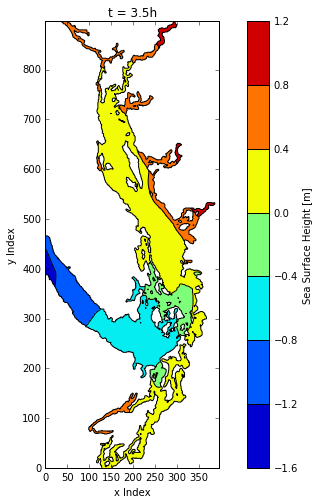With a few argument changes and additions we can plot contour bands on map coordinates. Note that viz_tools.plot_coastline() accepts either the file path/name of a bathymetry data file, or a nc.Dataset instance created from one. Here we do the latter because we also need the bathymetry latitudes and longitudes to pass into the contour plotting methods.

In :
grid = nc.Dataset('../../NEMO-forcing/grid/bathy_meter_SalishSea2.nc')
lats = grid.variables['nav_lat']
lons = grid.variables['nav_lon']

t = 3

fig, ax = plt.subplots(1, 1, figsize=(10, 8))
viz_tools.set_aspect(ax, coords='map', lats=lats)
contour_fills = ax.contourf(lons[:], lats[:], ssh3)
contour_lines = ax.contour(lons[:], lats[:], ssh3, colors='black', linestyles='solid')
viz_tools.plot_coastline(ax, grid, coords='map')
cbar = fig.colorbar(contour_fills)

ax.grid()
ax.set_xlabel('{longitude.long_name} [{longitude.units}]'.format(longitude=lons))
ax.set_ylabel('{latitude.long_name} [{latitude.units}]'.format(latitude=lats))
cbar.set_label('{label} [{units}]'.format(label=ssh.long_name.title(), units=ssh.units))
ax.set_title('t = {:.1f}h'.format(timesteps[t] / 3600))

Out:
<matplotlib.text.Text at 0x7f9076f60f98>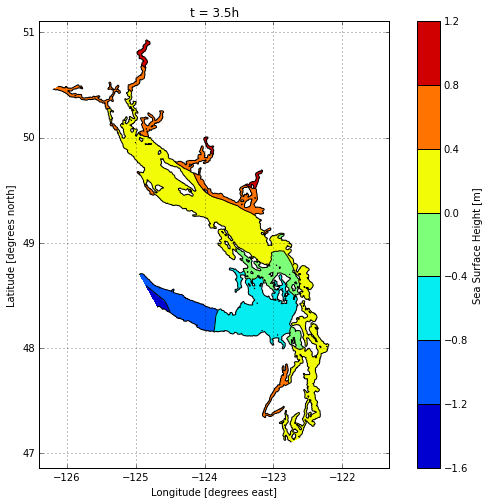## Plotting Temperature Field on Horizontal Planes¶

Now let's shift our attention to the temperature field:

In :
nc_tools.show_variable_attrs(tracers, 'votemper')

<class 'netCDF4._netCDF4.Variable'>
float32 votemper(time_counter, deptht, y, x)
units: degC
standard_name: temperature
_FillValue: 9.96921e+36
long_name: temperature
online_operation: ave(X)
interval_operation: 10.0
interval_write: 3600.0
coordinates: time_counter deptht nav_lat nav_lon
unlimited dimensions: time_counter
current shape = (24, 40, 898, 398)
filling off



In contrast to sea surface height, temperature results are 4-dimensional arrays with the axes ordered (t, z, y, x). The z dimension is positive downward (i.e. depth increases with increasing z-index values) as indicated by the positive: down attribute of the deptht variable;

In :
nc_tools.show_variable_attrs(tracers, 'deptht')

<class 'netCDF4._netCDF4.Variable'>
float32 deptht(deptht)
axis: Z
standard_name: model_level_number
units: m
positive: down
valid_min: 0.5
valid_max: 441.466
title: deptht
long_name: Vertical T levels
unlimited dimensions:
current shape = (40,)
filling off



The deptht variable values are the nominal midpoints of the model depth layers:

In :
tracers.variables['deptht'][:]

Out:
array([   0.5000003 ,    1.5000031 ,    2.50001144,    3.50003052,
4.50007057,    5.50015068,    6.50031042,    7.50062323,
8.50123596,    9.50243282,   10.50476551,   11.50931168,
12.51816654,   13.53541183,   14.56898212,   15.63428783,
16.76117325,   18.00713539,   19.48178482,   21.38997841,
24.10025597,   28.22991562,   34.68575668,   44.51772308,
58.48433304,   76.58558655,   98.06295776,  121.86651611,
147.08946228,  173.11448669,  199.57304382,  226.26029968,
253.06663513,  279.93453979,  306.834198  ,  333.75018311,
360.67453003,  387.60321045,  414.53408813,  441.46609497], dtype=float32)

Note that layers are approximately 1 m thick near the surface, but increase in thickness with depth. Furthermore, the model uses partial z-steps at depth, and it adjusts the surface layers in response to changes in sea surface level. So, the precise layer thicknesses and midpoint depths vary both temporally and spatially.

Aliasing the temperature and depth layer midpoint variables to save on keystrokes:

In :
temper = tracers.variables['votemper']
zlevels = tracers.variables['deptht']


As with sea surface height, we access the temperature field values using slices, but now we have 4 axes to deal with rather than 3. So:

• The surface layer (0 to ~1m) temperature field at the 3rd time step is temper[2, 0]
• The ~10.5m temperature field at time step zero is temper[0, 10]
• The ~2.5m temperature field at the last time step in a region that includes some of the Fraser River plume is:
In :
temper[-1, 2, 400:450, 275:325]

Out:
array([[ 10.89370728,  10.89942741,  10.91184521, ...,   0.        ,
0.        ,   0.        ],
[ 10.87751865,  10.88292503,  10.89572525, ...,   0.        ,
0.        ,   0.        ],
[ 10.86694431,  10.87142372,  10.8831234 , ...,   0.        ,
0.        ,   0.        ],
...,
[ 10.40889263,  10.3713665 ,  10.33268642, ...,   0.        ,
0.        ,   0.        ],
[ 10.42024708,  10.38743591,  10.35234833, ...,   0.        ,
0.        ,   0.        ],
[ 10.42642498,  10.40050793,  10.37171364, ...,   0.        ,
0.        ,   0.        ]], dtype=float32)

Apart from handling the presence of the z-axis dimension, plotting colour meshes of temperature field is very similar to what we have already done for sea surface height:

In :
t, zlevel = 0, 0

fig, ax = plt.subplots(1, 1, figsize=(10, 8))
viz_tools.set_aspect(ax)
cmap = plt.get_cmap('jet')
mesh = ax.pcolormesh(temper_tz, cmap=cmap)
cbar = fig.colorbar(mesh)
plt.axis((0, temper_tz.shape, 0, temper_tz.shape))

ax.grid()

ax.set_xlabel('x Index')
ax.set_ylabel('y Index')
cbar.set_label('{label} [{units}]'.format(label=temper.long_name.title(), units=temper.units))
ax.set_title(u't = {t:.1f}h, depth \u2248 {d:.2f}{z.units}'.format(t=timesteps[t] / 3600, d=zlevels[zlevel], z=zlevels))

Out:
<matplotlib.text.Text at 0x7f9077013dd8>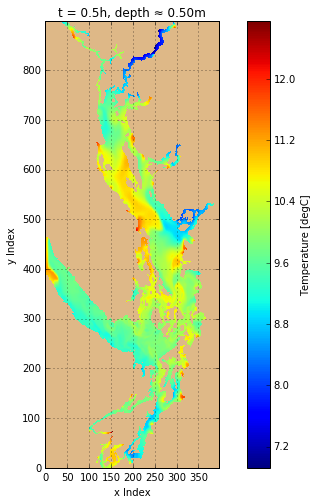Creating masked arrays (with the mask value set to zero) from time and depth slices of the temperature array allows us to create plots that show the land/water boundaries at depth.

In :
fig, axs = plt.subplots(1, 3, figsize=(16, 8), sharey=True)
for ax in axs:
viz_tools.set_aspect(ax)

cmap = plt.get_cmap('jet')

t = 0
levels = (0, 10, 26)
for ax, z in zip(axs, levels):
mesh = ax.pcolormesh(temper_tz, cmap=cmap, vmin=6, vmax=15)
cbar = fig.colorbar(mesh, ax=ax)
ax.set_title(u't = {t:.1f}h, depth \u2248 {d:.2f}{z.units}'.format(t=timesteps[t] / 3600, d=zlevels[z], z=zlevels))
ax.set_xlabel('x Index')
ax.grid()

axs.set_ylabel('y Index')
cbar.set_label('{label} [{units}]'.format(label=temper.long_name.title(), units=temper.units))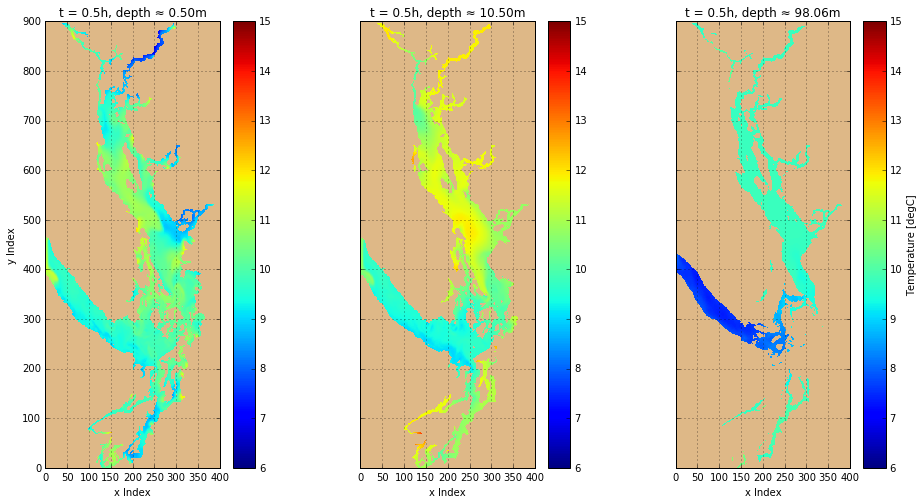## Adding Contour Lines to Colour Mesh Plots¶

Let's zoom in on an interesting looking area of the domain and create a colour mesh plot with temperature contour lines (isotherms):

In :
t, zlevel = 0, 0
y_slice = np.arange(425, 551)
x_slice = np.arange(200, 351)
temper_tz = np.ma.masked_values(temper[t, zlevel, y_slice, x_slice], 0)

fig, ax = plt.subplots(1, 1, figsize=(10, 8))
viz_tools.set_aspect(ax)
cmap = plt.get_cmap('jet')
mesh = ax.pcolormesh(x_slice, y_slice, temper_tz, cmap=cmap)
clines = ax.contour(
x_slice, y_slice, temper_tz, np.arange(8, 13),
colors=['black', 'black', 'red', 'black', 'black'])
ax.clabel(clines, fmt='%1.1f')
cbar = fig.colorbar(mesh, shrink=0.95)

ax.set_xlim(x_slice, x_slice[-1])
ax.set_ylim(y_slice, y_slice[-1])
ax.grid()

ax.set_xlabel('x Index')
ax.set_ylabel('y Index')
cbar.set_label('{label} [{units}]'.format(label=temper.long_name.title(), units=temper.units))
ax.set_title(u't = {t:.1f}h, depth \u2248 {d:.2f}{z.units}'.format(t=timesteps[t] / 3600, d=zlevels[zlevel], z=zlevels))

Out:
<matplotlib.text.Text at 0x7f9074499630>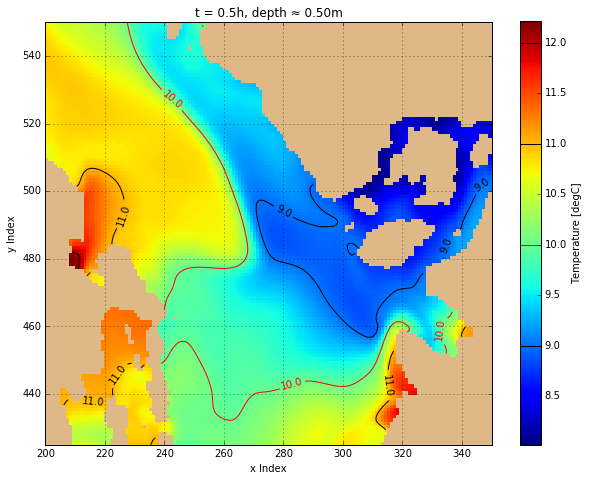## Anomaly Plots¶

It's often useful to plot a colour mesh of the differences in value of a field variable between a pair of time steps or runs to help to visualize changes over model time or changes due to alteration of model parameters, forcing, code, etc. As an example, we'll plot the temperature field at 2 consecutive time steps and the difference between them. For the difference field we'll use a colour map that is white at zero and increases in colour intensity for increasingly positive and negative values.

The viz_tools.calc_abs_max() function is useful to obtain the maximum absolute value in an array which can be used to set the normalization limits for the anomaly plots so that zero is in the centre of the colour range.

In :
help(viz_tools.calc_abs_max)

Help on function calc_abs_max in module salishsea_tools.viz_tools:

calc_abs_max(array)
Return the maximum absolute value in the array.

:arg array: Array to find the maximum absolute value of.
:type array: :py:class:numpy.ndarray or :py:class:netCDF4.Dataset

:returns: Maximum absolute value
:rtype: :py:class:numpy.float32


In :
fig, axs = plt.subplots(1, 3, figsize=(16, 8), sharey=True)
for ax in axs:
viz_tools.set_aspect(ax)

cmap = plt.get_cmap('jet')
anomaly_cmap = plt.get_cmap('bwr')
for c in (cmap, anomaly_cmap):

time_steps = (0, 2)
z = 0

# Temperature fields at the time steps
for ax, t in zip(axs[:2], time_steps):
mesh = ax.pcolormesh(temper_tz, cmap=cmap)
cbar = fig.colorbar(mesh, ax=ax)
ax.set_title(u't = {t:.1f}h, depth \u2248 {d:.2f}{z.units}'.format(t=timesteps[t] / 3600, d=zlevels[z], z=zlevels))
ax.set_xlabel('x Index')
ax.set_xlim(0, temper_tz.shape)
ax.set_ylim(0, temper_tz.shape)
ax.grid()
axs.set_ylabel('y Index')
cbar.set_label('{label} [{units}]'.format(label=temper.long_name.title(), units=temper.units))

# Temperature field difference between the time steps
ax = axs
temper_diff = temper[time_steps, z] - temper[time_steps, z]